# AND & OR Clauses in SQL

Consider the below query which retrieves the records borrower whose loan due date is in June.

```SELECT BRWR_ID, ISBN
FROM BOOK_LOAN
WHERE DUE_DATE >= ‘1-Jun-2015’;```

Above query also looks incomplete as seeing BRWR_ID and ISBN; one cannot identify the person who has borrowed or the book that he has borrowed. They are all IDs, which gives least information to the user. User will not understand what these IDs are about. Hence we need to show the names of borrower and book in above query instead of IDs. But we do not have names in BOOK_LOAN table. They are present in BORROWER and BOOKS tables respectively. Hence we need to query those tables too by linking BOOK_LOAN table. How do we link them to get the names? This is possible since all these three tables are mapped to each other by means of foreign keys. Here we need to join two tables with BOOK_LOAN to get the result. Hence we have two more conditions added to above query. We can link the conditions in the WHERE clause by using AND clause here. It makes sure all the conditions mentioned in the query are satisfied and shows the result. Hence we can write query like below to get meaningful result.

```SELECT BL.BRWR_ID, BL.ISBN
FROM BOOK_LOAN BL, BORROWER BR, BOOKS B
WHERE BL.BRWR_ID = BR.BRWR_ID
AND BL.ISBN = B.ISBN
AND BL.DUE_DATE >= '1-Jun-2015';```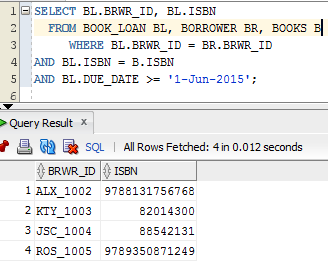Here note that the order of join condition would be first join the tables present in the FROM clause in the order they appear, and then have other filter conditions. But here again we are displaying the IDs which does not give meaningful result. Hence let us pull the correct columns from the respective tables.

```SELECT BR.BRWR_NAME, B.BOOK_NAME
FROM BOOK_LOAN BL, BORROWER BR, BOOKS B
WHERE BL.BRWR_ID = BR.BRWR_ID
AND BL.ISBN = B.ISBN
AND BL.DUE_DATE >= '1-Jun-2015';```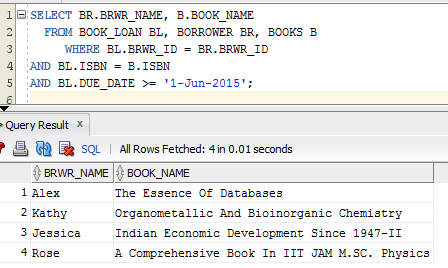Here query checks for the matching records from BOOK_COPY with both BORROWER and BOOKS, and gets the records. Then it filters based on the due date and displays only those columns requested in the SELECT query. Hence we get the result, which is correct and understandable to the user.

Sometimes users will have two conditions, and they would like to see the results, if any one of the condition is true. In such case OR condition is used to link the conditions in the WHERE clause. It will then display the results, when either of the condition is true.

Consider the query to pull the Book details which are received to the library in 2010 or in 2014. We have to query BOOK_COPY table to know when they have come to library.

```SELECT bc.ISBN, bc.COPY_NUM, bc.RECEIVED_DATE
FROM BOOK_COPY bc
WHERE TO_CHAR (bc.RECEIVED_DATE, 'YYYY') = '2010'
OR TO_CHAR (bc.RECEIVED_DATE, 'YYYY') = '2014';```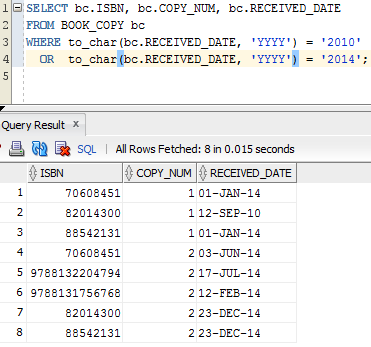### To make this query more accurate :

```SELECT bc.ISBN, b.BOOK_NAME, bc.COPY_NUM, bc.RECEIVED_DATE
FROM BOOK_COPY bc, BOOKS b
WHERE bc.ISBN = b.ISBN
AND (TO_CHAR (bc.RECEIVED_DATE, 'YYYY') = '2010'
OR TO_CHAR (bc.RECEIVED_DATE, 'YYYY') = '2014');```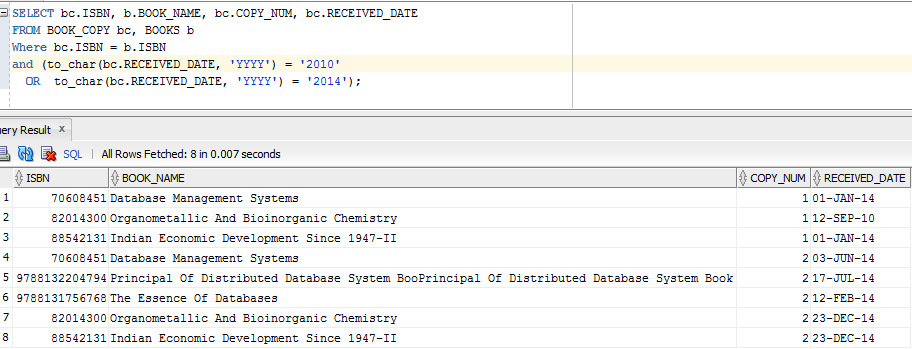Consider the query to pull the records whose loan date is in June 2015 or Due date is in June 2015. This needs to query BOOK_LOAN table for loan date and due date.

```SELECT BRWR_ID, ISBN, LOAN_DATE, DUE_DATE
FROM BOOK_LOAN BL
WHERE   BL.LOAN_DATE >= '1-Jun-2015' OR BL.DUE_DATE >= '1-Jun-2015';```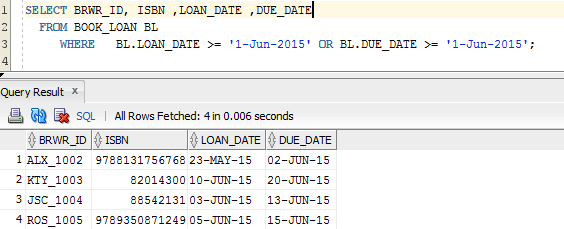But column details which user can understand can be got from their respective parent tables – BOOKS and BORROWER.

```SELECT BR.BRWR_NAME, B.BOOK_NAME, BL.LOAN_DATE, BL.DUE_DATE
FROM BOOK_LOAN BL, BORROWER BR, BOOKS B
WHERE BL.BRWR_ID = BR.BRWR_ID
AND BL.ISBN = B.ISBN
AND BL.LOAN_DATE >= '1-Jun-2015' OR BL.DUE_DATE >= '1-Jun-2015';```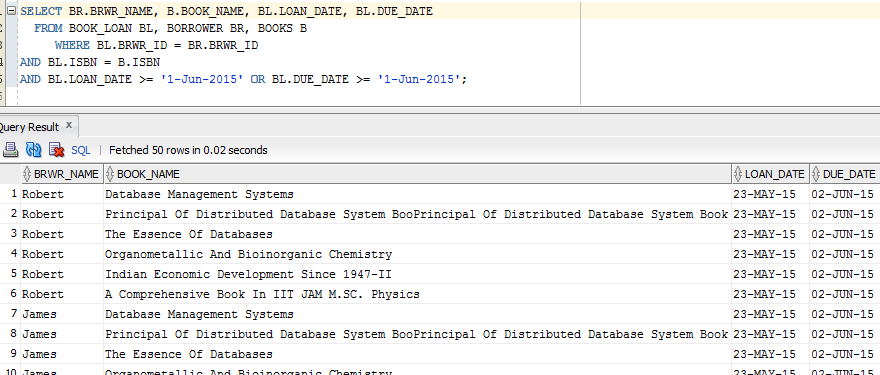What is wrong here? We were supposed to get only 4 records but we are getting more than 4 records! Above query will all the records which satisfies the BL.LOAN_DATE >= ‘1-Jun-2015’ OR BL.DUE_DATE >= ‘1-Jun-2015’ conditions, and then does the cartesian product with the BOOK_LOAN table. Hence it gave wrong result. Then what is wrong here ?

First we have to select all matching records from BOOKS, BORROWER and BOOK_LOAN, and then we need to check for loan date or due date. This can be done as below – braces are put for the OR condition as it needs to be executed on the result of matching records.

```SELECT BR.BRWR_NAME, B.BOOK_NAME
FROM BOOK_LOAN BL, BORROWER BR, BOOKS B
WHERE BL.BRWR_ID = BR.BRWR_ID
AND BL.ISBN = B.ISBN
AND (BL.LOAN_DATE >= '1-Jun-2015' OR BL.DUE_DATE >= '1-Jun-2015');```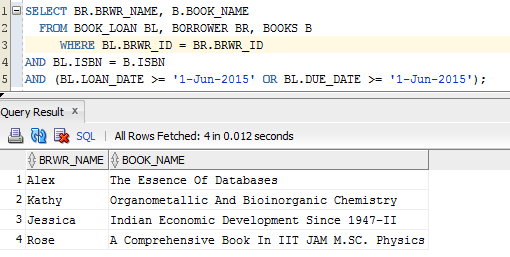Consider another example for OR clause: List the books written by James Martin or Ajai Kumar. Where do we get the details about author names? It’s in AUTHOR table. Where are the details about the books written by these authors? It’s in BOOK_AUTHOR table. Can we map these two tables? Yes we have the foreign keys and we can map them. Are these two tables sufficient to display the meaningful result? Both of these tables have IDs which users cannot understand. We need to show the book names to the user which is in the BOOKS table. Hence we need to query3 tables – AUTHOR, BOOK_AUTHOR and BOOKS.

```SELECT a.AUTHOR_NAME, b.BOOK_NAME
FROM AUTHOR a, BOOK_AUTHOR ba, BOOKS b
WHERE a.author_id = ba.author_id
AND ba.isbn = b.isbn
AND (a.author_name = ‘James Martin’ OR a.author_name = ‘Ajai Kumar’);```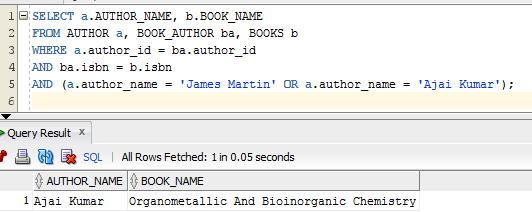This query shows only one record where as we have books written by both the authors. Why ?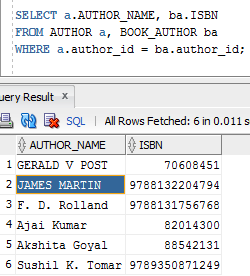What is wrong in our query? We had given the author name in lower case for ‘James Martin’, but it is in upper case. Hence we need to be careful while selecting the records based on the character for their cases. Hence our query will change to :

```SELECT a.AUTHOR_NAME, b.BOOK_NAME
FROM AUTHOR a, BOOK_AUTHOR ba, BOOKS b
WHERE a.author_id = ba.author_id
AND ba.isbn = b.isbn
AND (a.author_name = ‘JAMES MARTIN’ OR a.author_name = ‘Ajai Kumar’);```

Better way to write queries with character type is make it case insensitive.

```SELECT a.AUTHOR_NAME, b.BOOK_NAME
FROM AUTHOR a, BOOK_AUTHOR ba, BOOKS b
WHERE a.author_id = ba.author_id
AND ba.isbn = b.isbn
AND (UPPER (a.author_name)= ‘JAMES MARTIN’ OR UPPER (a.author_name)= = ‘AJAI KUMAR’);```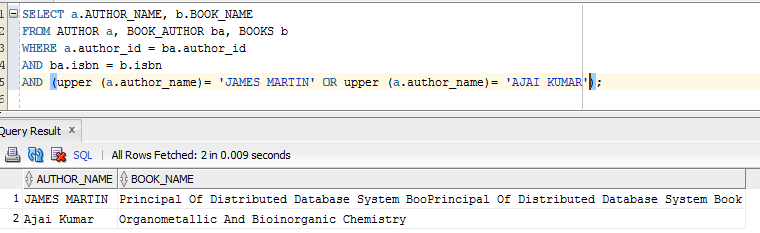Now the query gives the correct results.

Translate »